# Using Quadratic Equations to Represent Motion

##### Rating:
(9)
• (5)
• (0)
• (1)
• (0)
• (3)
Author: Sophia Tutorial
##### Description:

Solve a quadratic equation that represents the path of an object in motion.

(more)
Tutorial
what's covered
1. The Path of an Object in Motion
2. Calculating Maximum Height of an Object in Motion

# 1. The Path of an Object in Motion

Quadratic equations can be used to represent that path of objects as they go up in the air and come back down due to the force of gravity. Below is the graph of a volleyball that is being shot over a net, and at some distance from the net, it hits the ground. The curve is described by the following equation: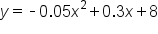,

where y equals the height of the ball, and x is the distance from the net.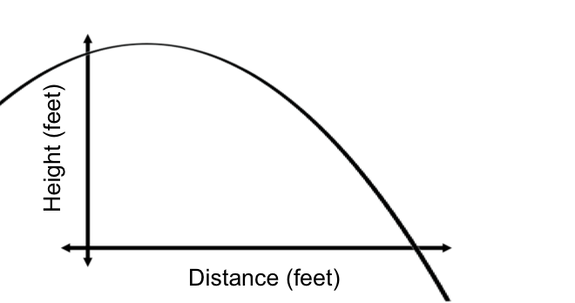We can imagine the x-axis of the graph as being the ground, and the y-axis of the graph is the volleyball net. And the curve itself is the path of the volley.

We would like to know at what distance the volleyball hits the ground. To answer this question, we need to find the value of x when y equals zero. There are a couple of methods to solving quadratic equations set equal to zero. One method is to factor the equation, to get an equation in the form 0 = (x + a)(x + b). Another method is to use the quadratic formula, which uses the coefficients of the equation in standard form (a, b, and c). We will use the quadratic formula to solve this equation, because by the looks of the coefficients in the equation, factoring is either going to be difficult or impossible.

Below is the worked out example using the quadratic formula: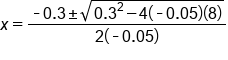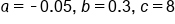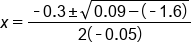Square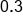and multiply 4 by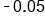and 8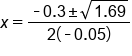Evaluate radical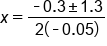Simplify the radical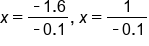Create two equations due to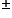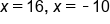Our solutions

We have two solutions here, one which is positive and one which is negative. Looking back at our diagram, we see that taking the negative answer doesn't make sense in this context. For this reason, we'll only take the positive value, which describes the distance from the net that the volleyball hits the ground.

hint
Always be sure to keep the context of the problem in mind. In the example above, we did not accept the negative solution, because negative distance didn't make sense in the context.

# 2. Calculating Maximum Height of an Object in Motion

Next, let's think about a volleyball being shot in the air in a vertical motion, once again going up, and eventually coming back down to the ground. The graph below shows the relationship between the height of the ball in relationship to time: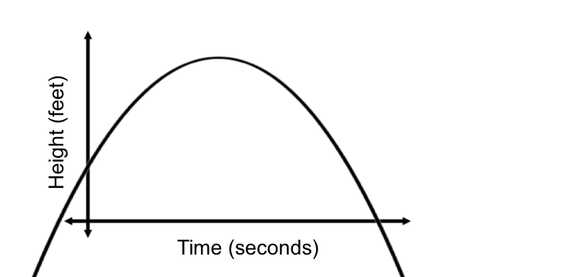We see that the x-axis represents time, measured in seconds, and the y-axis represents height, measured in feet.

The curve on the graph is described by the following equation: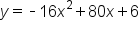The coefficients in this equation have important meanings within the context. -16x2 comes from the force of gravity on Earth, 80x represents the velocity of the volley ball (in this case, it is 80 ft/s), and 6 represents the height of the volleyball player hitting the ball.

We can use this quadratic equation to answer questions about the maximum height that the ball will reach, before it begins to come back down to the ground. Looking back at our graph, we notice that the maximum height of the volleyball is at the vertex of the parabola. How can we algebraically determine the coordinates of a vertex?

We use the following equation to find the x–coordinate of the vertex (which is also the equation to the axis of symmetry of the parabola):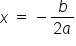, where a and b come from coefficients of the equation in standard form.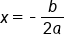Formula for axis of symmetry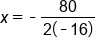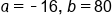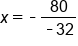Simplify denominator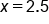Our solution

Our solved value for x represents the time at which the ball will reach the maximum height. We would like to calculate the height. To do this, we must plug this value of x back into the equation and find the associated y-value.Our quadratic equation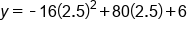x=2.5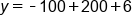Evaluate each term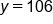Our solution

This means that after 2.5 seconds, the volleyball will reach a maximum height of 106 feet.

summary
A quadratic equation can be used to model the path of an object in motion, rising and falling due to gravity. If x represents time and y represents height, the quadratic equation and/or its graph can be used to answer questions about the object's path such as the time to travel a certain horizontal distance, or the height of the object at a certain time. We can use this to calculate maximum height of an object in motion by finding the vertex.

Rating Header